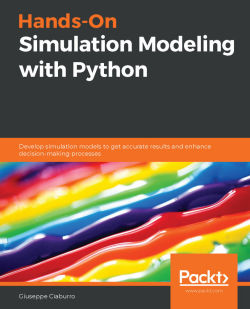# Hands-On Simulation Modeling with Python

By Giuseppe Ciaburro
• \$41.99 Print
• \$28.99 eBook
• \$12.99 eBook + Subscription
What do you get with a Packt Subscription?

• Constantly updated with 100+ new titles each month
• Breadth and depth in over 1,000+ technologies

Simulation modeling helps you to create digital prototypes of physical models to analyze how they work and predict their performance in the real world. With this comprehensive guide, you'll understand various computational statistical simulations using Python.

Starting with the fundamentals of simulation modeling, you'll understand concepts such as randomness and explore data generating processes, resampling methods, and bootstrapping techniques. You'll then cover key algorithms such as Monte Carlo simulations and Markov decision processes, which are used to develop numerical simulation models, and discover how they can be used to solve real-world problems. As you advance, you'll develop simulation models to help you get accurate results and enhance decision-making processes. Using optimization techniques, you'll learn to modify the performance of a model to improve results and make optimal use of resources. The book will guide you in creating a digital prototype using practical use cases for financial engineering, prototyping project management to improve planning, and simulating physical phenomena using neural networks.

By the end of this book, you'll have learned how to construct and deploy simulation models of your own to overcome real-world challenges.

Publication date:
July 2020
Publisher
Packt
Pages
346
ISBN
9781838985097

# Section 1: Getting Started with Numerical Simulation

In this section, the basic concepts of simulation modeling are addressed. This section helps you to understand the fundamental concepts and elements of numerical simulation.

This section contains the following chapters:

Chapter 1, Introducing Simulation Models

Chapter 2, Understanding Randomness and Random Numbers

Chapter 3, Probability and Data Generating Processes